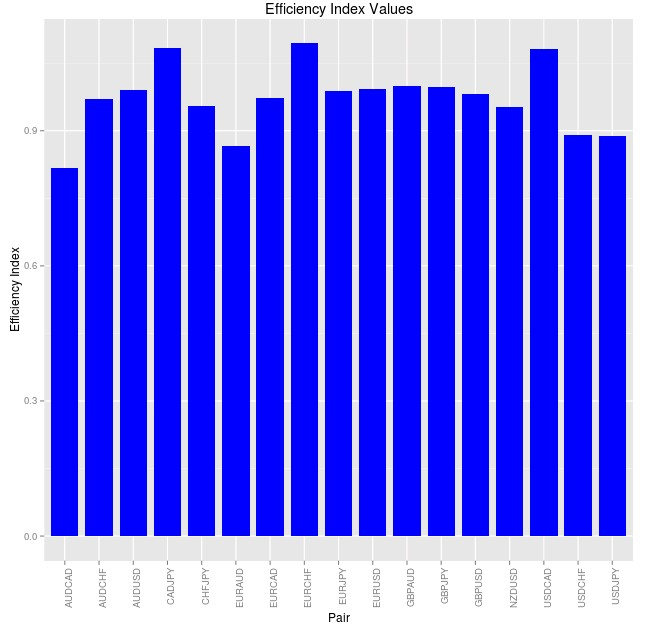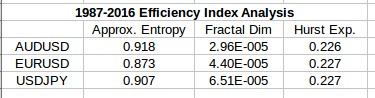## Using R in Trading: Calculating a simple Efficiency Index

On my last post I discussed the results of a recent paper that used an efficiency index calculation in order to compare the relative efficiency of different gold/currency pairs. Since the measuring of the relative efficiency of currency pairs is very interesting – particularly for long term data – I decided to attempt to replicate a similar measurement using R to allow for the easy calculation of this index for currency pairs. Within this post I will walk you through the way in which I have implemented this measurement and why it is fundamentally different from the measurements used within the aforementioned paper. We will talk about ways in which the measurement can be improved and what information it presently gives us about currency pairs.  We will also see how the results differ very significantly from those we would expect due to the paper and we’ll talk about why this is the case. To run the code below you will need to install the pracma, quantmod and fractaldim libraries within your R setup.

To implement a similar calculation to the one showed within the article I decided to create an Efficiency Index using the Hurst exponent, the fractal dimension and the approximate entropy. The key requirement to create an Efficiency Index is that all the statistics included should be well bounded and there should be a clear expected value for a fully efficient case, a clear expected value for a random series. In the case of the Hurst exponent the expected value is 0.5 and the exponent is bounded between 0 and 1, for the fractal dimension the expected value is 1.5 and the value is bounded between 1 and 2 but – as it’s also mentioned within the article – for the approximate entropy things get a little bit trickier. In order to bound the approximate entropy value I simply resorted to the creation of a random distribution and I used the entropy of this series as the maximum possible entropy value for a random series. Since entropy goes from 0 to a maximum cap for a random series this benchmark was used for the calculation of the entropy contribution.

The calculate_EI function I have created – showed in the code above – takes a time series and then calculates the Efficiency Index plus the contribution from each separate efficiency index component and returns them as a dataframe. The Efficiency Index is simply the square root of the sum of the squares of all the differences between actual and random case values divided by the range for each different component. It in essence represents a “distance” between your measured efficiency level and the value of the same measurement for a perfectly random case. Getting data for the past 500 days for 17 pairs using the QuantMod getSymbol function, which queries data from Oanda, we can obtain the Efficiency index values shown below.As you can probably notice differences between the efficiency index values for the different pairs are not very big with almost all pairs having a distance between 0.8 and 1.2. The most inefficient pair according to the index within this period is the EURCHF while the most efficient is the AUDCAD. The EURUSD ranks around the middle of the list while the USDJPY is among the most efficient. However all pairs have a very large distance from the ideal random case, showing that all pairs are rather inefficient when compared with a random walk. Granted the distance are much larger than those within the article and this stems from a fundamental difference in the way that the Efficiency Index is calculated, which can explain the rather odd results that we get in terms of the efficiency ranking between the different pairs.

A fundamental problem with the above calculation is that a random walk may appear to have non-random behavior at any time interval shorter than infinity, that is, there is always a chance that by mere luck a random walk looks like something that is not random. In order to correct for this problem the article uses bootstrapping with replacement to generate random time series that are used to create a benchmark efficiency index from which the “true” EI difference with an expected benchmark can be measured. That is, we also need to calculate whether our EI distance is truly relevant or just an expected and merely apparent deviation from randomness at the sample length we are investigating. Implementing this sampling and evaluation of the EI over random time series of the same length would provide a potentially significant improvement to the above calculation.I have also performed the same Efficiency Index analysis using long term time series 30+ years for the EUR/USD, USD/JPY and AUD/USD. While for shorter term time series the fractal dimension component is always a significant component of the series – contributing from 20 to 50% of the overall value – when we go into 30 years the fractal dimension contribution immediately drops to something minuscule (less than 0.01% in all cases). Entropy becomes the largest contributor at this point (always contributing something above the 90% mark and the efficiency index tends to equalize among the pairs. When looking at the EUR/USD, USD/JPY and AUD/USD data from 1987 the overall differences in the indexes between the pairs is less than 3%. The fractal dimension always contributes something close to zero and the differences in approximate entropy tend to create the differences in the efficiency index while the Hurst exponent contribution remains almost fixed through the different series. The table above shows the overall contribution to the index of the three different values (square of difference divided by range) for the three studied symbols over the 1987-2016 period (daily rates).

The results obtained so far are not very revealing since the values for all symbols tend to be rather similar, especially at very long evaluation periods. This analysis fails to explain why the finding of inefficiencies is much easier on the EUR/USD and so difficult on the AUD/USD and leaves the door open to further experimentation. Of course several improvements – as I have mentioned above – are possible and I will continue to explore them to see if I can find a way to gain further resolution in the analysis. If you would like to learn more about automated trading and how you too can learn how to find and trade real historical inefficiencies in the Forex market please consider joining Asirikuy.com, a website filled with educational videos, trading systems, development and a sound, honest and transparent approach towards automated trading.